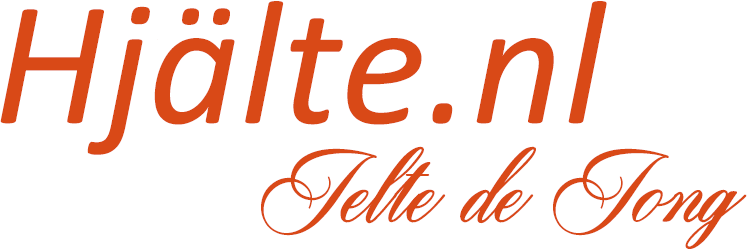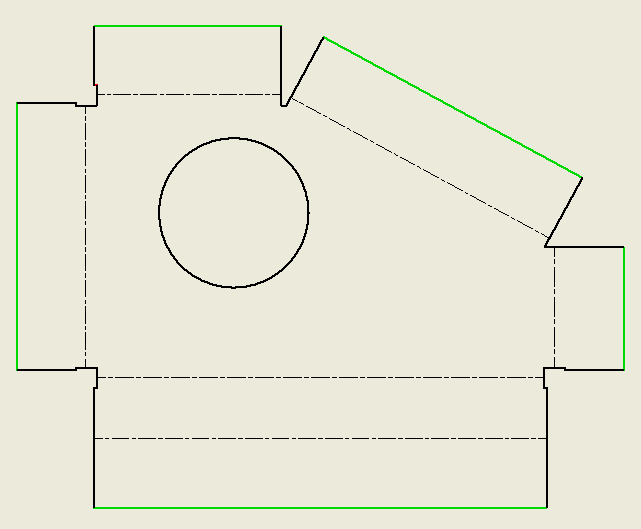Writing a rule that will generate the dimensions for all bend lines in a sheet metal flat pattern drawing view is not easy. This is a rule that I wanted to write for a long time but did not manage to do till now. The problem is not finding the bend lines nor finding all contour lines. But the problem is how more finding a contour line that is parallel with a bend line and is also useful.

For example, consider the following view. As you can see I can find multiple outer contour lines that could be used for dimensioning the bend line. But I think only 1 is useful for a dimension.If I have a look at my total flat view then only need to consider the green lines from the contour.I struggled to filter out those lines. But then I remembered this Google code contest question. (That I did not solve at the time...)

 Google code jam 2015: Round 1A - Problem C. Logging A certain forest consists of N trees, each of which is inhabited by a squirrel.The boundary of the forest is the convex polygon of smallest area which contains every tree, as if a giant rubber band had been stretched around the outside of the forest.Formally, every tree is a single point in two-dimensional space with unique coordinates (Xi,Yi), and the boundary is the convex hull of those points.Some trees are on the boundary of the forest, which means they are on an edge or a corner of the polygon. The squirrels wonder how close their trees are to being on the boundary of the forest.One at a time, each squirrel climbs down from its tree, examines the forest, and determines the minimum number of trees that would need to be cut down for its own tree to be on the boundary. It then writes that number down on a log.Determine the list of numbers written on the log.

It might seem unrelated but to start solving this problem you need to find all trees that are on the edge of the forest and are toughed by the "rubber band". For my view, I need to find only the points/lines that are touged by the "rubber band". So i did some digging and found a algoritme that would give me the points that i needed. With that information i could write my rule. The rest of the rule is mostly about creating and filtering lists. The moral of the story: participate in coding competitions you might learn something that you don't need at the time but later can be useful.

Anyway, try this rule. I can think of situations where it will not work but I think it would be a cool challenge for anyone to find a better solution.

``````Public Class ThisRule
Public Sub Main()

Dim doc As DrawingDocument = ThisDoc.Document
Dim sheet As Sheet = doc.ActiveSheet
Dim view As DrawingView = ThisApplication.CommandManager.Pick(
SelectionFilterEnum.kDrawingViewFilter,
"Select a drawing view")
Dim curves As IEnumerable(Of DrawingCurve) = view.DrawingCurves.Cast(Of DrawingCurve).ToList()

Dim bendCurves = curves.
Where(Function(curve) curve.EdgeType = DrawingEdgeTypeEnum.kBendDownEdge Or
curve.EdgeType = DrawingEdgeTypeEnum.kBendUpEdge).ToList()

Dim contourCurves = curves.
Where(Function(curve)
Return curve.EdgeType = DrawingEdgeTypeEnum.kUnknownEdge And
(curve.CurveType = CurveTypeEnum.kLineCurve Or curve.CurveType = CurveTypeEnum.kLineSegmentCurve)
End Function).ToList()

Dim points = contourCurves.Select(Function(curve) curve.StartPoint).ToList()
Dim endPoints = contourCurves.Select(Function(curve) curve.EndPoint).ToList()

Dim hull As List(Of Point2d) = GetConvexHull(points)

Dim hullCurves As New List(Of DrawingCurve)
For Each curve As DrawingCurve In contourCurves
If (ContainsCurve(hull, curve)) Then
End If
Next

Dim trans = ThisApplication.TransactionManager.StartTransaction(doc, "Add fold dimensions.")

Dim directions = hullCurves.Select(Function(c) Vector2Angle(c.Segments.Item(1).Geometry.Direction)).Distinct().ToList()
For Each direction As Double In directions
Dim directedBends = bendCurves.
Where(Function(c) DoubleForEquals.Equals(Vector2Angle(c.Segments.Item(1).Geometry.Direction), direction)).
ToList()
If (directedBends.Count = 0) Then Continue For

Dim directedCountors = hullCurves.
Where(Function(c) DoubleForEquals.Equals(Vector2Angle(c.Segments.Item(1).Geometry.Direction), direction)).
ToList()
If (directedCountors.Count = 0) Then Continue For

Dim ordedList As New List(Of DrawingCurve)
Dim lastCurve As DrawingCurve = directedCountors.First()

While directedBends.Count <> 0
Dim minDistance = Double.MaxValue
Dim closestCurve As DrawingCurve = Nothing
For Each curve As DrawingCurve In directedBends
Dim d As Double = ThisApplication.MeasureTools.GetMinimumDistance(lastCurve, curve)
If (d < minDistance) Then
minDistance = d
closestCurve = curve
End If
Next
directedBends.Remove(closestCurve)
lastCurve = closestCurve
End While

If directedCountors.Count > 1 Then
End If

Dim biggest = CreateDimensions(sheet, ordedList)

If directedCountors.Count > 1 Then
biggest.Delete()
End If
Next

trans.End()
End Sub

Public Function CreateDimensions(sheet As Sheet, list As List(Of DrawingCurve)) As GeneralDimension
Dim biggest As GeneralDimension = Nothing

For i = 0 To list.Count - 2
Dim curve1 = list.Item(i)
Dim curve2 = list.Item(i + 1)

Dim intent1 = sheet.CreateGeometryIntent(curve1)
Dim intent2 = sheet.CreateGeometryIntent(curve2)

Dim xMin1 = Math.Min(curve1.StartPoint.X, curve1.EndPoint.X)
Dim xMax2 = Math.Max(curve2.StartPoint.X, curve2.EndPoint.X)

Dim yMin1 = Math.Min(curve1.StartPoint.Y, curve1.EndPoint.Y)
Dim yMax2 = Math.Max(curve2.StartPoint.Y, curve2.EndPoint.Y)

Dim x = (xMin1 + xMax2) / 2
Dim y = (yMin1 + yMax2) / 2

Dim p As Point2d = ThisApplication.TransientGeometry.CreatePoint2d(x, y)
Dim dimension = sheet.DrawingDimensions.GeneralDimensions.AddLinear(p, intent1, intent2, DimensionTypeEnum.kAlignedDimensionType)

If (biggest Is Nothing) Then
biggest = dimension
ElseIf (biggest.ModelValue < dimension.ModelValue) Then
biggest = dimension
End If
Next
Return biggest
End Function

Public Function Vector2Angle(vector As UnitVector2d) As Double
Dim o As Double
If (vector.Y <= 0 And vector.X <= 0) Then
o = Math.Abs(vector.Y) / Math.Abs(vector.X)
Else
o = vector.Y / vector.X
End If

Return Math.Round(Math.Tanh(o), 7)
End Function

Public Function ContainsCurve(points As List(Of Point2d), curve As DrawingCurve)
Dim startFound = False
Dim endFound = False
For Each point As Point2d In points
If (ComparePoint(curve.StartPoint, Point)) Then
startFound = True
End If
If (ComparePoint(curve.EndPoint, Point)) Then
endFound = True
End If
Next
Return (startFound And endFound)
End Function
Public Function ComparePoint(v1 As Point2d, v2 As Point2d)
If (DoubleForEquals.IsEqual(v1.X, v2.X) And DoubleForEquals.IsEqual(v1.Y, v2.Y)) Then
Return True
Else
Return False
End If
End Function

Public Shared Function cross(ByVal O As Point2d, ByVal A As Point2d, ByVal B As Point2d) As Double
Return (A.X - O.X) * (B.Y - O.Y) - (A.Y - O.Y) * (B.X - O.X)
End Function

Public Shared Function GetConvexHull(ByVal points As List(Of Point2d)) As List(Of Point2d)
If points Is Nothing Then Return Nothing
If points.Count() <= 1 Then Return points
Dim n As Integer = points.Count(), k As Integer = 0
Dim H As List(Of Point2d) = New List(Of Point2d)(New Point2d(2 * n - 1) {})
points.Sort(Function(a, b) If(a.X = b.X, a.Y.CompareTo(b.Y), a.X.CompareTo(b.X)))

Dim i As Integer = 0
For i = 0 To n - 1

While k >= 2 AndAlso cross(H(k - 2), H(k - 1), points(i)) <= 0
k -= 1
End While

H(Math.Min(System.Threading.Interlocked.Increment(k), k - 1)) = points(i)
Next

i = n - 2
Dim t As Integer = k + 1

While i >= 0

While k >= t AndAlso cross(H(k - 2), H(k - 1), points(i)) <= 0
k -= 1
End While

H(Math.Min(System.Threading.Interlocked.Increment(k), k - 1)) = points(i)
i -= 1
End While

Return H.Take(k - 1).ToList()
End Function
'
' This code was written by Jelte de Jong, and published on www.hjalte.nl
'
' Permission Is hereby granted, free of charge, to any person obtaining a copy of this
' software And associated documentation files (the "Software"), to deal in the Software
' without restriction, including without limitation the rights to use, copy, modify, merge,
' publish, distribute, sublicense, And/Or sell copies of the Software, And to permit persons
' to whom the Software Is furnished to do so, subject to the following conditions:
'
' The above copyright notice And this permission notice shall be included In all copies Or
' substantial portions Of the Software.
'
' THE SOFTWARE Is PROVIDED "AS IS", WITHOUT WARRANTY Of ANY KIND, EXPRESS Or IMPLIED,
' INCLUDING BUT Not LIMITED To THE WARRANTIES Of MERCHANTABILITY, FITNESS For A PARTICULAR
' PURPOSE And NONINFRINGEMENT. In NO Event SHALL THE AUTHORS Or COPYRIGHT HOLDERS BE LIABLE
' For ANY CLAIM, DAMAGES Or OTHER LIABILITY, WHETHER In AN ACTION Of CONTRACT, TORT Or
' OTHERWISE, ARISING FROM, OUT Of Or In CONNECTION With THE SOFTWARE Or THE USE Or OTHER
' DEALINGS In THE SOFTWARE.
End Class``````

# Skills:

Autodesk Inventor, Vault, Git, C#, vb, .net, php HTML, css, js

# Education:

University computer science.
HBO Mechanical engineer.
MBO Fine mechanics.

# Experience:

Programmer and Mechanical engineer at Kelvion
(2016 - 20..)

Mechanical engineer at Strukton
(2009 - 2016)

Mechanical engineer at RDG-engineering
(2007 - 2009)

CNC Programmer at VMC
(2005 - 2007)

volunteer at Taizé
(2007)

# Certifications:

Objectgeoriënteerd analyseren en ontwerpen, Objectgeoriënteerd programmeren in Java, Webapplicaties: de clientkant, Databases, Security Aware Programmer, Web Security Specialist